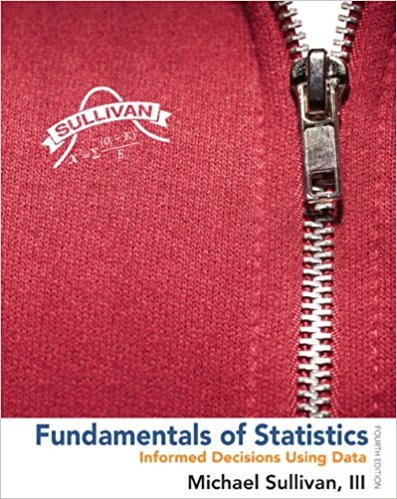×
Get Full Access to Fundamentals Of Statistics - 4 Edition - Chapter 3.4 - Problem 21ayu
Get Full Access to Fundamentals Of Statistics - 4 Edition - Chapter 3.4 - Problem 21ayu

×

# Solved: SMART Car The following data represent the milesISBN: 9780321838704 30

## Solution for problem 21AYU Chapter 3.4

Fundamentals of Statistics | 4th Edition

• Textbook Solutions
• 2901 Step-by-step solutions solved by professors and subject experts
• Get 24/7 help from StudySoup virtual teaching assistantsFundamentals of Statistics | 4th Edition

4 5 1 242 Reviews
19
0
Problem 21AYU

SMART Car The following data represent the miles per gallon of a random sample of SMART cars with a three cylinder, 1.0-liter engine.

 31.5 36 37.8 38.4 40.1 42.3 34.3 36.3 37.9 38.8 40.6 42.7 34.5 37.4 38 39.3 41.4 43.5 35.5 37.5 38.3 39.5 41.5 47.5

Source: www.fueleconomy.gov

(a)  Compute the z-score corresponding to the individual who obtained 36.3 miles per gallon. Interpret this result.

(b)  Determine the quartiles.

(c)  Compute and interpret the interquartile range, IQR.

(d)  Determine the lower and upper fences. Are there any outliers?

Step-by-Step Solution:

Step 1 of 5

Given Information:

The given data is:

 31.5 36 37.8 38.4 40.1 42.3 34.3 36.3 37.9 38.8 40.6 42.7 34.5 37.4 38 39.3 41.4 43.5 35.5 37.5 38.3 39.5 41.5 47.5

Sample size, n = 24

__________________________

Step 2 of 5

Step 3 of 5

##### ISBN: 9780321838704

This textbook survival guide was created for the textbook: Fundamentals of Statistics, edition: 4. Fundamentals of Statistics was written by and is associated to the ISBN: 9780321838704. This full solution covers the following key subjects: compute, smart, interpret, gallon, determine. This expansive textbook survival guide covers 51 chapters, and 2146 solutions. The answer to “?SMART Car The following data represent the miles per gallon of a random sample of SMART cars with a three cylinder, 1.0-liter engine.31.536.037.838.440.142.334.336.337.938.840.642.734.537.438.039.341.443.535.537.538.339.541.547.5 Source: www.fueleconomy.gov(a) Compute the z-score corresponding to the individual who obtained 36.3 miles per gallon. Interpret this result.(b) Determine the quartiles.(c) Compute and interpret the interquartile range, IQR.(d) Determine the lower and upper fences. Are there any outliers?” is broken down into a number of easy to follow steps, and 61 words. The full step-by-step solution to problem: 21AYU from chapter: 3.4 was answered by , our top Statistics solution expert on 04/03/17, 08:02AM. Since the solution to 21AYU from 3.4 chapter was answered, more than 11915 students have viewed the full step-by-step answer.

## Discover and learn what students are asking

Statistics: Informed Decisions Using Data : Assessing Normality
?A ______ ______ ______is a graph that plots observed data versus normal scores

Statistics: Informed Decisions Using Data : Inference about the Difference between Two Medians: Dependent Samples
?In Problems 3–10, use the Wilcoxon matched-pairs signedranks test to test the given hypotheses at the a = 0.05 level of significance. The dependent sa

Unlock Textbook Solution